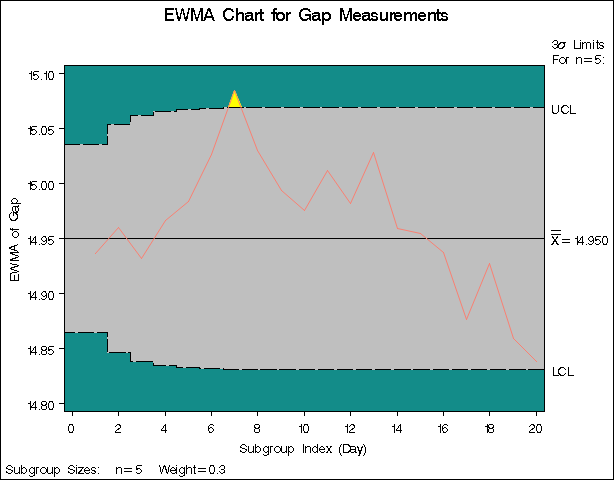#### Creating EWMA Charts from Subgroup Summary Data

See MACEW1 in the SAS/QC Sample LibraryThe previous example illustrates how you can create EWMA charts using raw data (process measurements). However, in many applications the data are provided as subgroup summary statistics. This example illustrates how you can use the EWMACHART statement with data of this type.

The following data set (`Clipsum`) provides the data from the preceding example in summarized form:

```data Clipsum;
input Day GapX GapS;
GapN=5;
datalines;
1  14.904  0.18716
2  15.014  0.09317
3  14.866  0.25006
4  15.048  0.23732
5  15.024  0.26792
6  15.126  0.12260
7  15.220  0.23098
8  14.902  0.17254
9  14.910  0.19824
10  14.932  0.24035
11  15.096  0.25618
12  14.912  0.16903
13  15.138  0.15928
14  14.798  0.26329
15  14.944  0.20876
16  14.896  0.09965
17  14.734  0.22512
18  15.046  0.24141
19  14.702  0.17880
20  14.788  0.16634
;
```

A partial listing of `Clipsum` is shown in Figure 9.4. There is exactly one observation for each subgroup (note that the subgroups are still indexed by `Day`). The variable `GapX` contains the subgroup means, the variable `GapS` contains the subgroup standard deviations, and the variable `GapN` contains the subgroup sample sizes (these are all five).

Figure 9.4: The Summary Data Set `Clipsum`

 The Data Set Clipsum

Day GapX GapS GapN
1 14.904 0.18716 5
2 15.014 0.09317 5
3 14.866 0.25006 5
4 15.048 0.23732 5
5 15.024 0.26792 5

You can read this data set by specifying it as a HISTORY= data set in the PROC MACONTROL statement, as follows:

```options nogstyle;
goptions ftext=swiss;
symbol color=salmon h=0.8;
title 'EWMA Chart for Gap Measurements';
proc macontrol history=Clipsum;
ewmachart Gap*Day / weight=0.3
cframe = vibg
cinfill = ligr
coutfill = yellow
cconnect = salmon;
run;
options gstyle;
```

The NOGSTYLE system option causes ODS styles not to affect traditional graphics. Instead, the GOPTIONS and SYMBOL statements and EWMACHART statement options control the appearance of the graph. The GSTYLE system option restores the use of ODS styles for traditional graphics produced subsequently. The resulting EWMA chart is shown in Figure 9.5.

Note that `Gap` is not the name of a SAS variable in the data set but is, instead, the common prefix for the names of the three SAS variables `GapX`, `GapS`, and `GapN`. The suffix characters X, S, and N indicate mean, standard deviation, and sample size, respectively. Thus, you can specify three subgroup summary variables in a HISTORY= data set with a single name (`Gap`), which is referred to as the process. The variables `GapX`, `GapS`, and `GapN` are all required. The name `Day` specified after the asterisk is the name of the subgroup-variable.

Figure 9.5: EWMA Chart from Summary DataIn general, a HISTORY= input data set used with the EWMACHART statement must contain the following variables:

• subgroup variable

• subgroup mean variable

• subgroup standard deviation variable

• subgroup sample size variable

Furthermore, the names of subgroup mean, standard deviation, and sample size variables must begin with the process name specified in the EWMACHART statement and end with the special suffix characters X, S, and N, respectively. If the names do not follow this convention, you can use the RENAME option in the PROC MACONTROL statement to rename the variables for the duration of the MACONTROL procedure step (see Creating Charts for Means and Ranges from Summary Data for an example of the RENAME option).

In summary, the interpretation of process depends on the input data set.

• If raw data are read using the DATA= option (as in the previous example), process is the name of the SAS variable containing the process measurements.

• If summary data are read using the HISTORY= option (as in this example), process is the common prefix for the names of the variables containing the summary statistics.